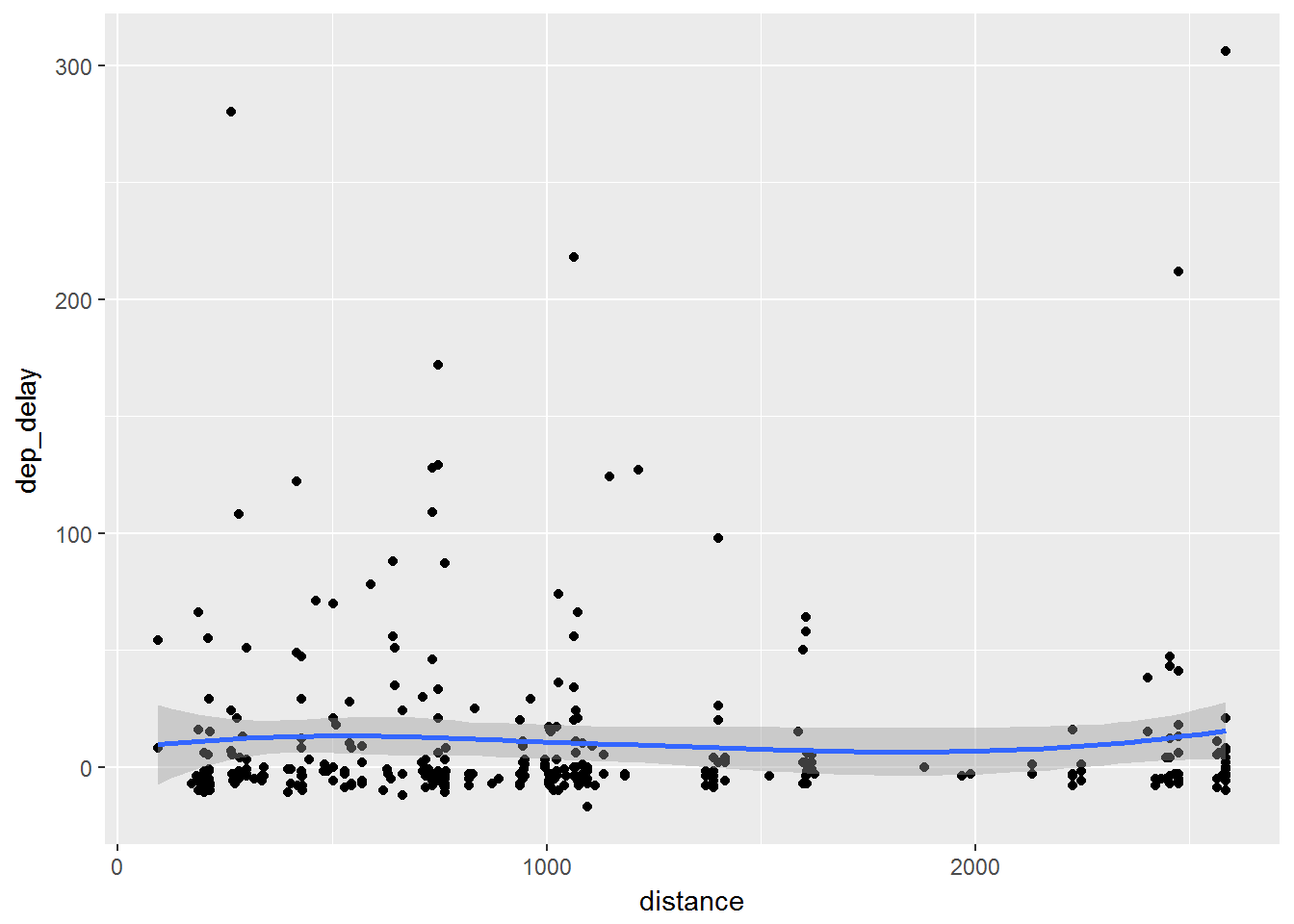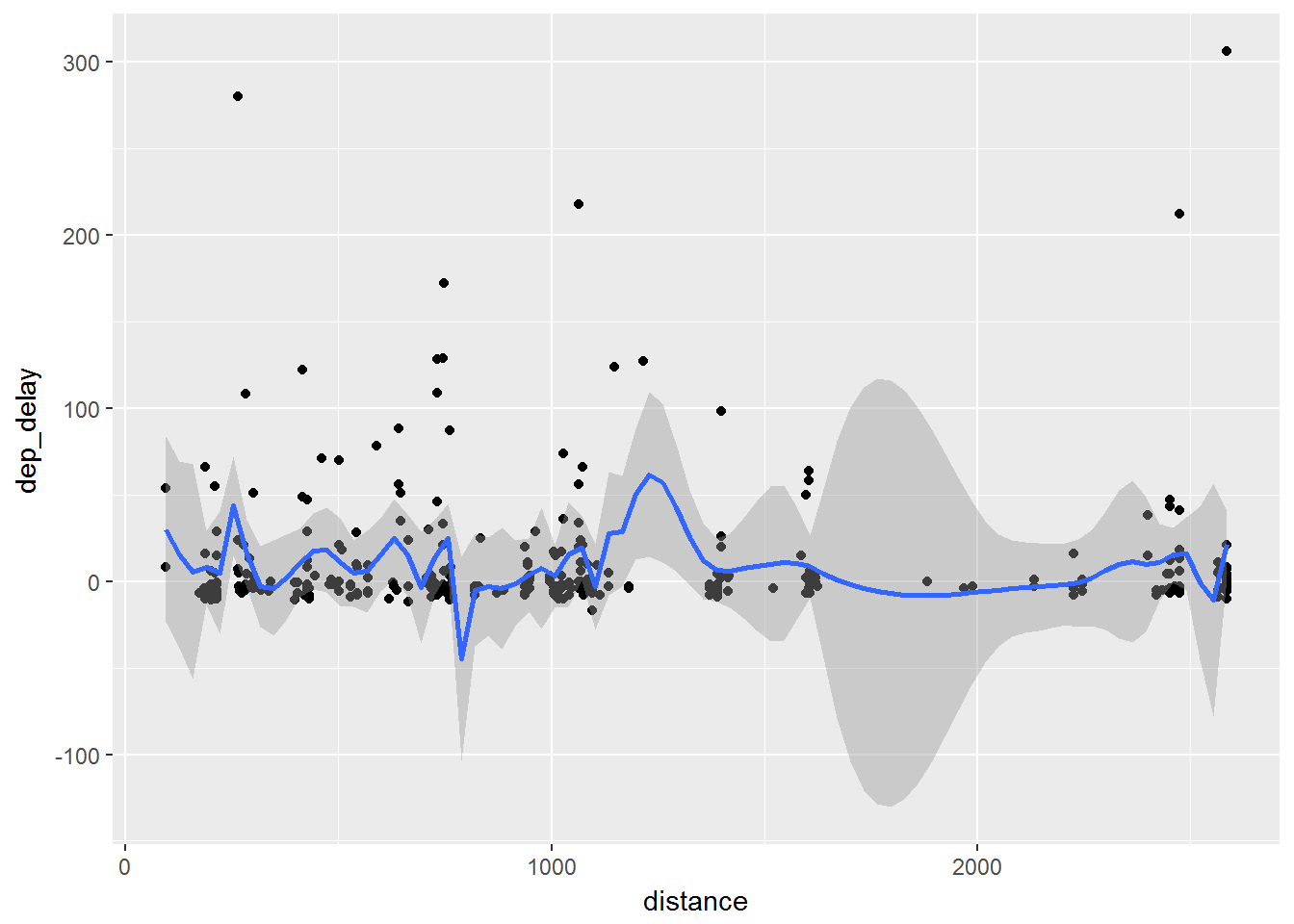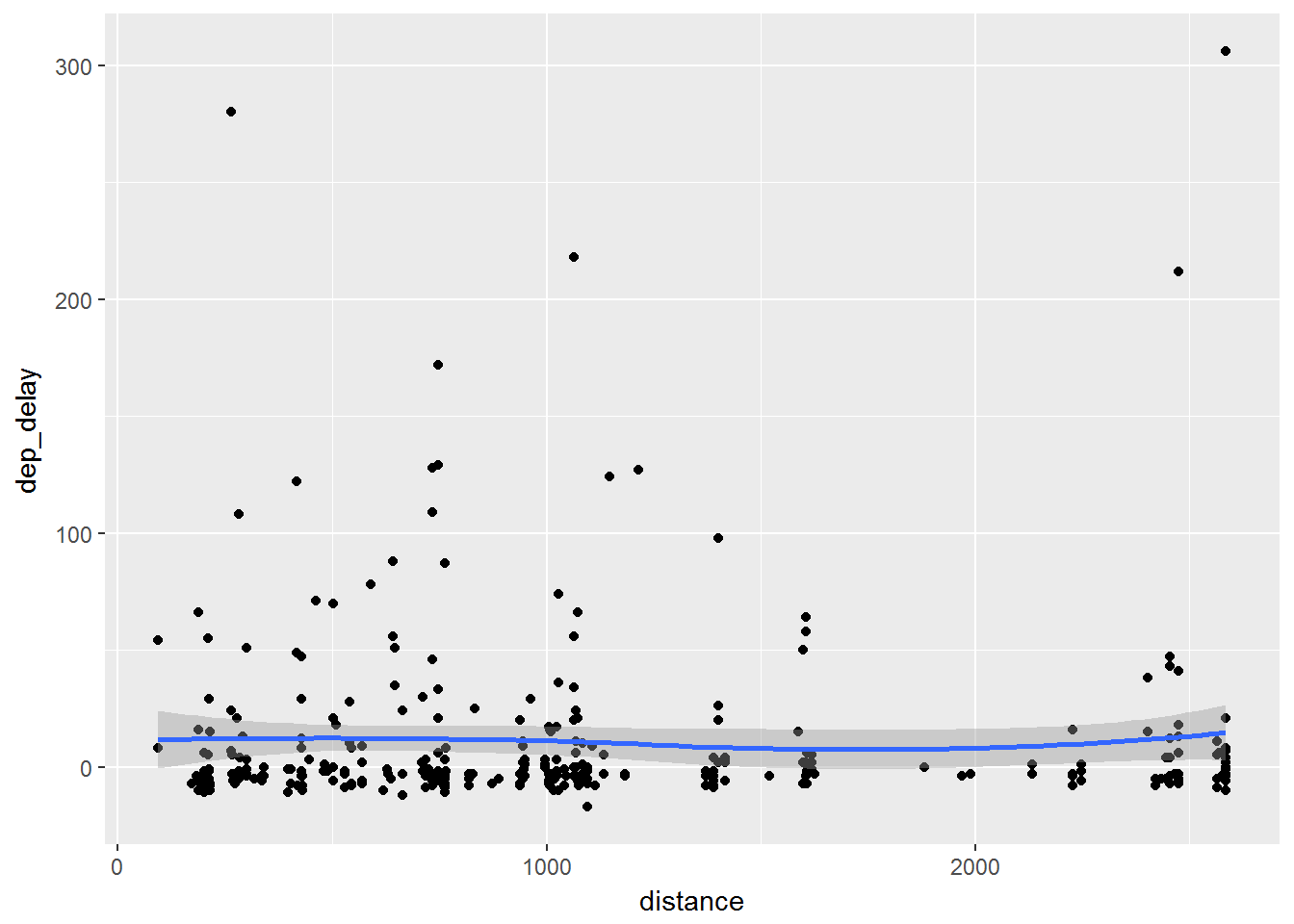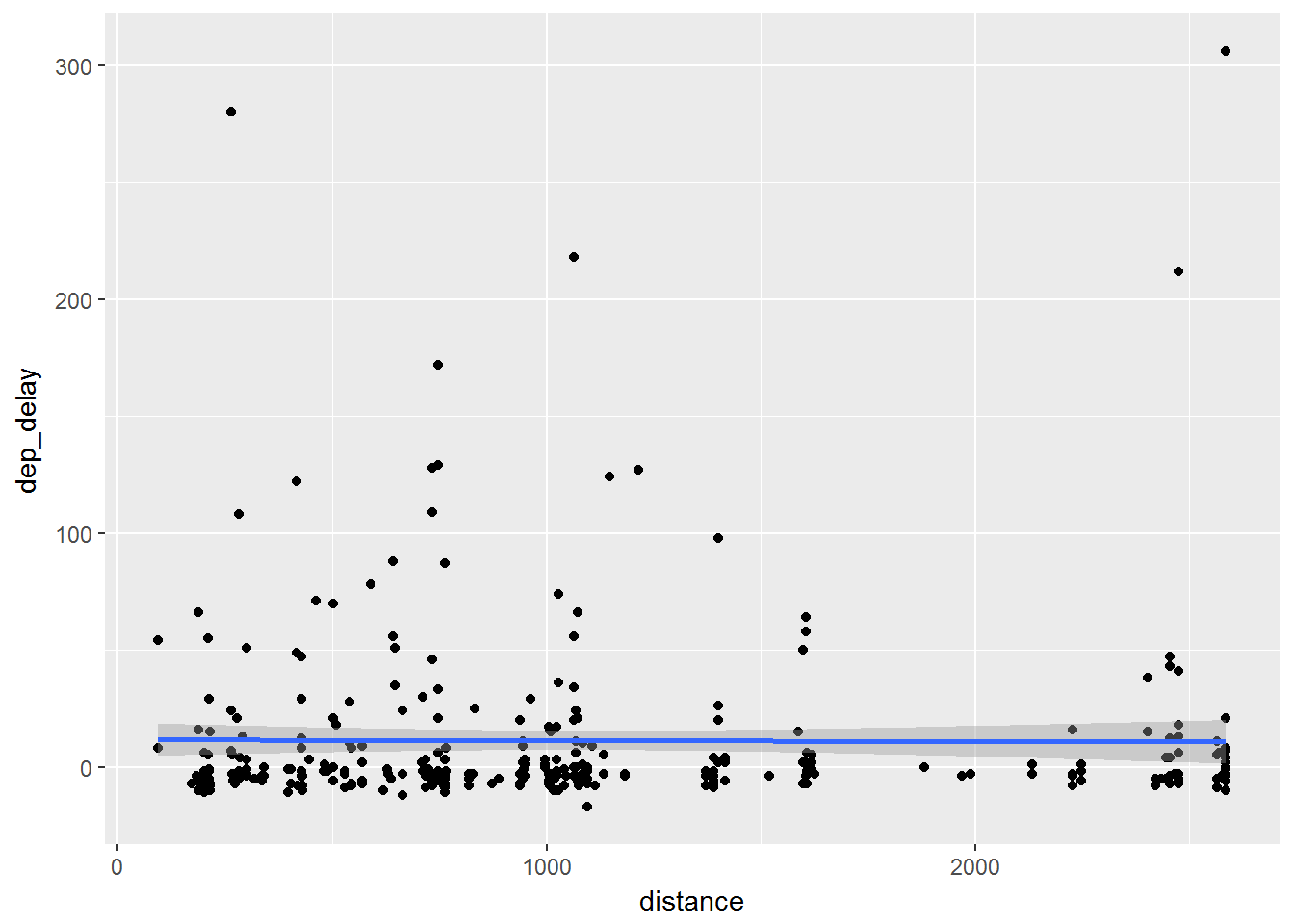# Smoothing

It can be hard to view trends with just points alone. Many times we wish to add a smoothing line in order to see what the trends look like. This can be especially helpful when trying to understand regressions.

We will take out scatter plot and apply a smoothing line to this:

``````ggplot(data, aes(x=distance, y= dep_delay)) +
geom_point() +
geom_smooth()``````

Again, the smoothing line comes after our points which means it is another layer added onto our graph:Note that the `geom_smooth()` function adds confidence bands on the smooth as well. We can remove these by adding `se=FALSE` inside the `geom_smooth()` function:

``````ggplot(data, aes(x=distance, y= dep_delay)) +
geom_point() +
geom_smooth(se=FALSE)``````

This produces the following plot:Consider what happens when you switch the layers around.

1. Graph just the data step:

``ggplot(data, aes(x=distance, y= dep_delay))``

2. Then add just the smooth

``````ggplot(data, aes(x=distance, y= dep_delay)) +
geom_smooth()``````

3. Finally add the points in:

``````ggplot(data, aes(x=distance, y= dep_delay)) +
geom_smooth() +
geom_point()``````

Note what happens as you slowly build these layers. This is a major part of the power of `ggplot2`

## Varying the Smooth

We have so far just seen how to add the smooth without being able to do anything but add or subtract the confidence bands. We now will change the smoothness of our smooth that we added. To do so we add `span=__` inside the `geom_smooth()` layer:

``````ggplot(data, aes(x=distance, y= dep_delay)) +
geom_point() +
geom_smooth(span = 0.1)
ggplot(data, aes(x=distance, y= dep_delay)) +
geom_point() +
geom_smooth(span = 1)``````

Above shows the coding for 2 possibilities of these changes to the smooth.Note that with `span = 0.1` we have a more rough smoothing than we had previously.When we changed the `span = 1` we can see that this is much smoother. The `span` can be varied from 0 to 1, where 0 is very rough and 1 is very smooth.

## Different Types of Smooths

There are different types of smooths that we can do. We will consider:

`loess`

`gam`

### Loess Smooths

Loess smoothing is a process by which many statistical softwares do smoothing. In `ggplot2` this should be done when you have less than 1000 points, otherwise it can be time consuming.

``````ggplot(data, aes(x=distance, y= dep_delay)) +
geom_point() +
geom_smooth(method="loess")``````

As you can see with the code we just add `method="loess"` into the `geom_smooth()` layer.### `gam` Smoothing

`gam` smoothing is called generalized additive mode smoothing. It works with a large number of points. We specify this by adding `method="gam", formula = y~s(x)` into the `geom_smooth()` layer.

``````library(mgcv)
ggplot(data, aes(x=distance, y= dep_delay)) +
geom_point() +
geom_smooth(method="gam", formula = y ~s(x))``````

The code for this is very similar and we can see how it looks below: